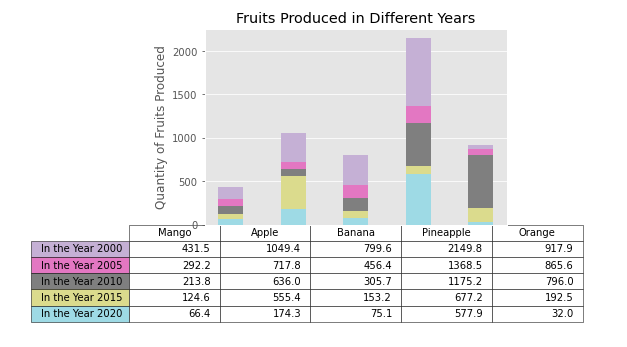# Matplotlib Bar Plot – Complete Tutorial For Beginners## Introduction

In this tutorial, we will be learning about how we can build Bar Plot by using Matplotlib library for your machine learning or data science projects. We will discuss various types of bar charts that have different features and useful information. Throughout this matplotlib barplot tutorial, you’ll learn about the details that should be taken care of while working with bar charts. Finally, we’ll also see how bar plots can be combined with other plots like pie charts using matplotlib library itself.

### Importing Matplotlib Library

Before beginning with this matplotlib bar plot tutorial, we’ll first have to import Matplotlib Library along with some other python libraries for our examples.

In :
```import matplotlib.pyplot as plt
import numpy as np
import pandas as pd
```

## Matplotlib Bar Plot

A bar plot or bar chart is a graph that represents the category of data with rectangular bars with lengths and heights that is proportional to the values which they represent. The bar plots can be plotted horizontally or vertically.

### Syntax of Bar Plot

matplotlib.pyplot.bar(x, height, width=0.8, bottom=None, *, align=’center’, data=None, **kwargs)

• x: sequence of scalars – This parameter provides the x-coordinates of the bars
• height: scalar or sequence of scalars – This helps in setting the height of the bars
• width: scalar or array-like, optional – This sets the width of the bars
• bottom : scalar or array-like, optional – Through this parameter the ‘y-coordinates’ of the bars bases is set.
• align : {‘center’, ‘edge’}, optional, default: ‘center’ – Using this we can position the bar plot.
NOTE: For aligning the plot on right side, you have to pass negative width and pass the align parameter value as “edge”

The function returns a container with the desired bars and sometimes errorbars are also visualized.

### Example 1: Simple Bar Plot

This first example of bar chart tutorial shows a simple example of how we can build a bar chart. The %matplotlib inline help in setting the backend of matplotlib to the ‘inline’ backend of the frontends like Jupyter Notebook.

The plt.style.use(‘ggplot’) is passed to emulate the style of ggplot function in matplotlib. Basically ggplot function is used in R library. With the help of the enumerate function, we are plotting multiple values in the bar plot with their associated values.

Lastly, after calling matplotlib’s bar function and passing relevant parameters, we can set the x-axis and y-axis labels. You may find that x-axis labels are congested, causing difficulty in comprehending, to solve this we have used the rotation parameter of xticks function that helps in rotating the x-axis labels.

The last command passed is plt.show(), this function helps in starting an event loop. It checks for all the currently active figure objects, opens them in interactive windows for appropriate displays.

NOTE: You may find that your plot is visualized without the usage of plt.show() command, this function basically performs lot of hidden operations with the GUI of your system. There would be cases when not using this function will produce an error. Thus, it should always be used.

In :
```%matplotlib inline
plt.style.use('ggplot')

x = ['Apple', 'Banana', 'Mango', 'WaterMelon', 'Pineapple', 'Grapes']
quantity = [5, 6, 15, 22, 24, 8]

x_pos = [i for i, _ in enumerate(x)]

plt.bar(x_pos, quantity, color='yellow')
plt.xlabel("Fruits")
plt.ylabel("Quantity of Fruits")
plt.title("The Quantity of the Fruits Grown in Kg")

plt.xticks(x_pos, x,rotation = 45)

plt.show()
```
Output: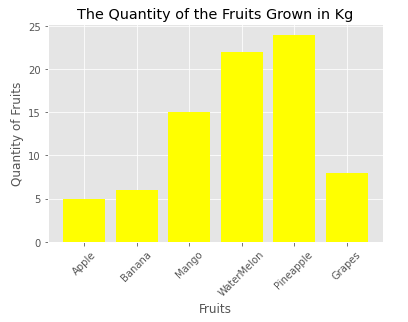### Example 2: Stacked Bar Plot with Errorbars

The 2nd type of bar plot is the one where we can stack two different bar plots and create a stacked bar chart. This kind of plot is helpful in comparing two different sets of information. Here the stacked bar chart also depicts the error bars in the final visualization.

The data for the stacked bar plot is random mean and standard deviation data for different groups of Men and Women. We also specify the width of the bars for the plot.

Other functions like ylabel, title, xticks, yticks, and legend are used for setting other important features of bar plot.

In :
```N = 8
menMeans = (40, 35, 30, 35, 27,34, 20, 25)
womenMeans = (35, 28,36, 40, 20, 33, 21, 30)
menStd = (2, 3, 4, 5, 3, 5, 2, 4)
womenStd = (3, 5, 2, 3, 3, 4, 4, 2)
ind = np.arange(N)
width = 0.35

p1 = plt.bar(ind, menMeans, width, yerr=menStd)
p2 = plt.bar(ind, womenMeans, width,
bottom=menMeans, yerr=womenStd)

plt.ylabel('Scores')
plt.title('Scores by group and gender')
plt.xticks(ind, ('G1', 'G2', 'G3', 'G4', 'G5', 'G6', 'G7', 'G8'))
plt.yticks(np.arange(0, 81, 10))
plt.legend((p1, p2), ('Boys', 'Girls'))

plt.show()
```
Output: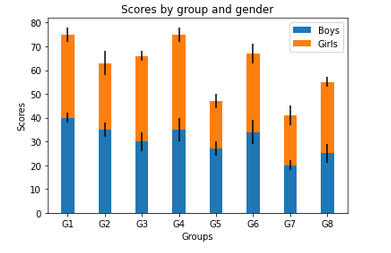### Example 3: Grouped Bar Chart with labels and gridlines

Coming to the 3rd example of this matplotlib based bar plot tutorial, we’ll be looking at how we can build a horizontal grouped bar chart. In the previous example, we saw stacked bar chart, the same chart can be made into a horizontal bar plot with the help of barh function of matplotlib library.

Once we have randomly obtained the labels and data, we only have to pass these values into the barh function. We’ll call subplots function for plotting this horizontal bar function.

For better comprehension of the values that bars of the plot convey, we are calling the grid() function. This will help in creating gridlines over the plot.

At last, tight layout function is called for displaying the plot in a proper manner in the given space.

Output: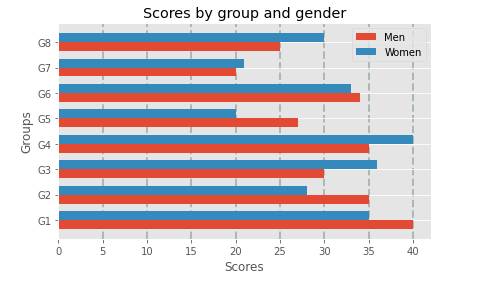### Example 4: Donut Chart/Pie Chart using Matplotlib’s Bar Function

A very interesting example of this tutorial, here we will be plotting pie chart/ donut chart by using matplotlib’s bar function. Here first we have to generate random data, then the data is normalized before using it for plotting.

After this, we call the colormap attribute to assign the colors to the donut chart. The next step is to pass data to bar function.

In :
```fig, ax = plt.subplots(subplot_kw=dict(polar=True))

size = 0.3
vals = np.array([[60., 32.], [37., 40.], [29., 10.]])
#normalize vals to 2 pi
valsnorm = vals/np.sum(vals)*2*np.pi
#obtaining the ordinates of the bar edges
valsleft = np.cumsum(np.append(0, valsnorm.flatten()[:-1])).reshape(vals.shape)

cmap = plt.get_cmap("gist_rainbow_r")
outer_colors = cmap(np.arange(50)*10)
inner_colors = cmap(np.arange(80)*100)

ax.bar(x=valsleft[:, 0],width=valsnorm.sum(axis=1), bottom=1-size, height=size,color=outer_colors, edgecolor='w', linewidth=1, align="edge")

ax.bar(x=valsleft.flatten(),width=valsnorm.flatten(), bottom=1-2*size, height=size,color=inner_colors, edgecolor='w', linewidth=1, align="edge")

ax.set(title="Pie Plot/Donught Chart with `ax.bar` and polar coordinates")
ax.set_axis_off()
plt.show()
```
Output: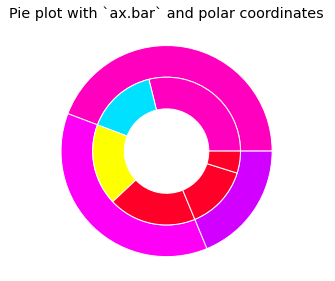### Example 5: Bar Plot on Polar Axis

Generally, bar plots are built on rectangular axes but in this case, we are building bar plot on polar axis. Initially, we generate random data, then the position of the bar is specified using linspace() function. The radii, width, and colors of bars are assigned using random function of numpy.

With the help of the subplot function, we will be plotting the bar plot over polar axis.

In :
```np.random.seed(20981815)

# Computing pie slices
N = 20
theta = np.linspace(0.0, 2 * np.pi, N, endpoint=False)
width = np.pi / 4 * np.random.rand(N)

ax = plt.subplot(111, projection='polar')
ax.bar(theta, radii, width=width, bottom=0.0, color=colors, alpha=0.5)

plt.show()
```
Output: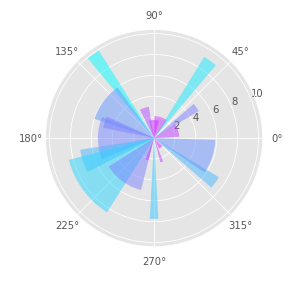### Example 6: Bar Plot on the basis of a Table

This last example of bar plot tutorial using matplotlib, we will be learning how we can build bar chart with the help of tabular data. For this plot, we first have to generate random data, then rows and columns for the table are created.

We then set the color of the bar plot. The next step is to plot the bars and add text lables according to the table.

Finally, we add the table by calling matplotlib’s table function. The layout of the table is adjusted for easier comprehension of both the plot and the table.

As the plot is depicting the growth of fruits in five different years, a single bar consists of 5 different shades that represent the 5 different years. The table displaying the years along with fruits produced in kg in different years.

In :
```data = [[ 66386, 174296,  75131, 577908,  32015],
[ 58230, 381139,  78045,  99308, 160454],
[ 89135,  80552, 152558, 497981, 603535],
[ 78415,  81858, 150656, 193263,  69638],
[139361, 331509, 343164, 781380,  52269]]

columns = ('Mango', 'Apple', 'Banana', 'Pineapple', 'Orange')
rows = ['In the Year %d' % x for x in (2000, 2005, 2010, 2015, 2020)]

values = np.arange(0, 2500, 500)
value_increment = 1000

# Setting the color of the plot
colors = plt.cm.tab20_r(np.linspace(0, 0.5, len(rows)))
n_rows = len(data)

index = np.arange(len(columns)) + 0.3
bar_width = 0.4

# Initializing the vertical-offset
y_offset = np.zeros(len(columns))

# Plot bars and create text labels for the table
cell_text = []
for row in range(n_rows):
plt.bar(index, data[row], bar_width, bottom=y_offset, color=colors[row])
y_offset = y_offset + data[row]
cell_text.append(['%1.1f' % (x / 1000.0) for x in y_offset])

# Reversing colors and text labels to display the last value at the top.
colors = colors[::-1]
cell_text.reverse()

# For adding table at the bottom of bar chart
the_table = plt.table(cellText=cell_text,rowLabels=rows,rowColours=colors,colLabels=columns,loc='bottom')
the_table.set_fontsize(10)
the_table.scale(1.5, 1.5)

# Adjust layout to make room for the table: